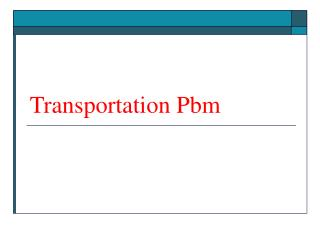# Transportation Pbm - PowerPoint PPT PresentationDownload PresentationTransportation Pbm

Transportation Pbm
Download Presentation## Transportation Pbm

- - - - - - - - - - - - - - - - - - - - - - - - - - - E N D - - - - - - - - - - - - - - - - - - - - - - - - - - -
##### Presentation Transcript

1. Transportation Pbm

2. Transportation Problem • The Transportation Model • Solution of a Transportation Problem • North west corner solution • Least cost solution

3. The Transportation Model Characteristics • A product is transported from a number of sources to a number of destinations at the minimum possible cost. • Each source is able to supply a fixed number of units of the product, and each destination has a fixed demand for the product. • The linear programming model has constraints for supply at each source and demand at each destination. • All constraints are equalities in a balanced transportation model where supply equals demand. • Constraints contain inequalities in unbalanced models where supply does not equal demand.

4. Example of a Transportation Model

5. Example of a Transportation Model - Problem: how many tons of wheat to transport from each grain elevator to each mill on a monthly basis in order to minimize the total cost of transportation ? - Data: Grain ElevatorSupplyMill Demand 1. Kansas City 150 A. Chicago 200 2. Omaha 175 B. St.Louis 100 3. Des Moines 275 C. Cincinnati 300 Total 600 tons Total 600 tons

6. 3 DCs and 3 customers. You have 3 DCs, and need to deliver product to 3 customers. Find cheapest way to satisfy all demand? CH 200 KC 150 St 100 O 175 DM 275 CI 300

7. Transportation Model ExampleLP Model Formulation minimize Z = 6x1A + 8x1B + 10x1C + 7x2A + 11x2B + 11x2C + 4x3A + 5x3B + 12x3C subject to x1A + x1B + x1C = 150 x2A + x2B + x2C = 175 x3A + x3B+ x3C = 275 x1A + x2A + x3A = 200 x1B + x2B + x3B = 100 x1C + x2C + x3C = 300 xij  0 where xij = tons of wheat from each grain elevator, i, i = 1, 2, 3, to each mill j, j = A,B,C Network of transportation routes for wheat shipments

8. Solution of the Transportation ModelTableau Format • Transportation problems are solved manually within a tableau format. • Each cell in a transportation tableau is analogous to a decision variable that indicates the amount allocated from a source to a destination. The Transportation Tableau

9. Solution of the Transportation ModelSolution Methods • Transportation models do not start at the origin where all decision values are zero; they must instead be given an initial feasible solution. • Initial feasible solution determination methods include: • - northwest corner method • - Lowest cost method • - Vogel’s Approximation Method • Methods for solving the transportation problem itself include: • - Transportation Algorithm.